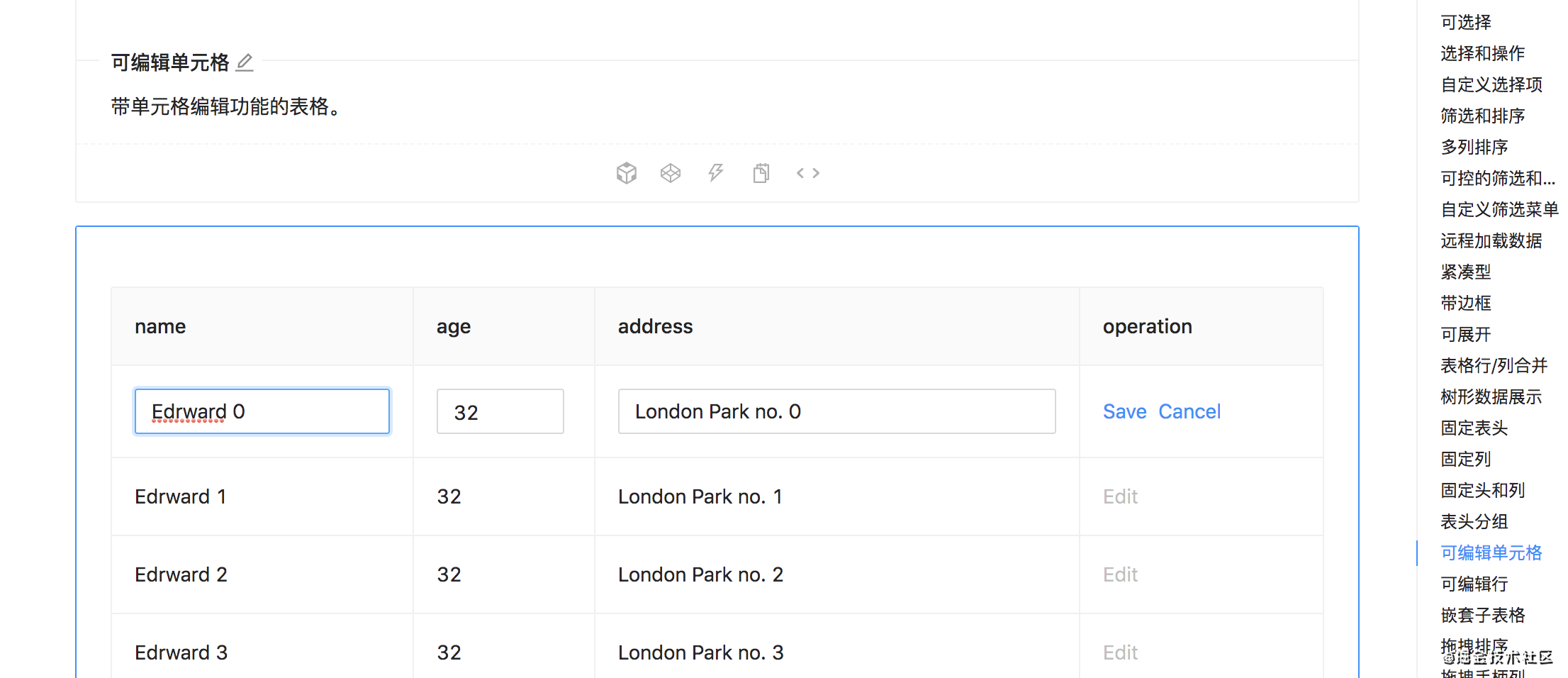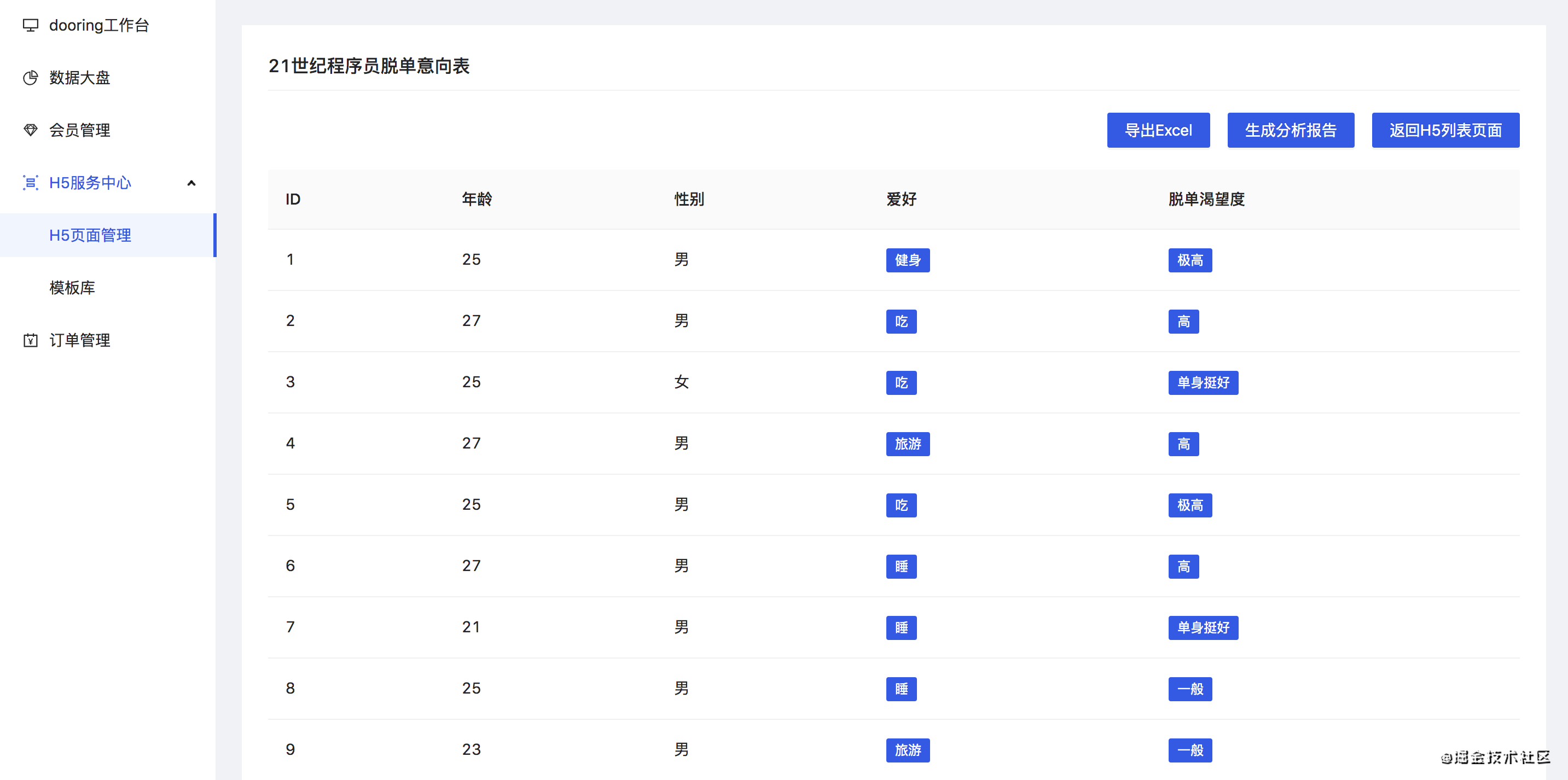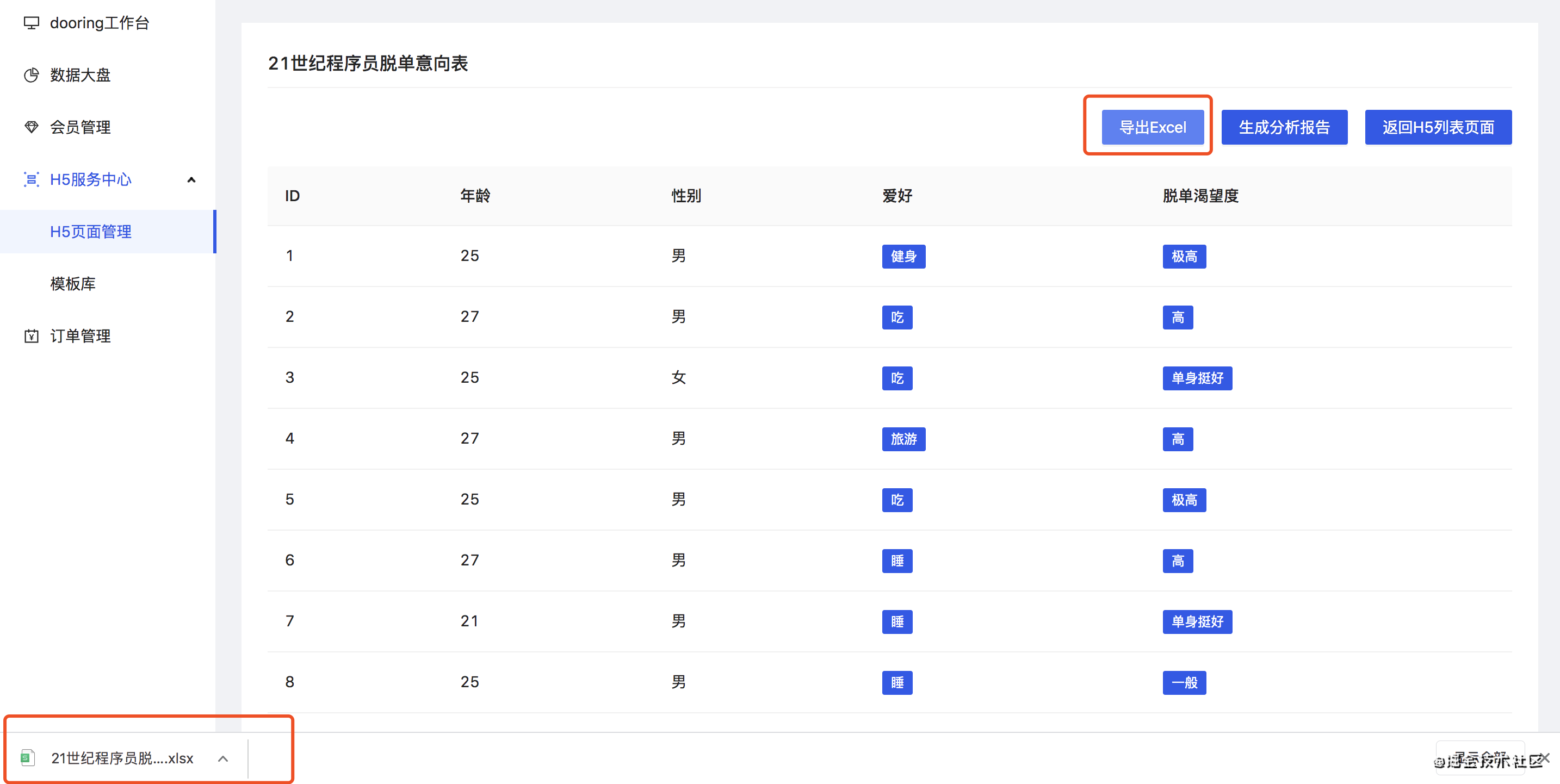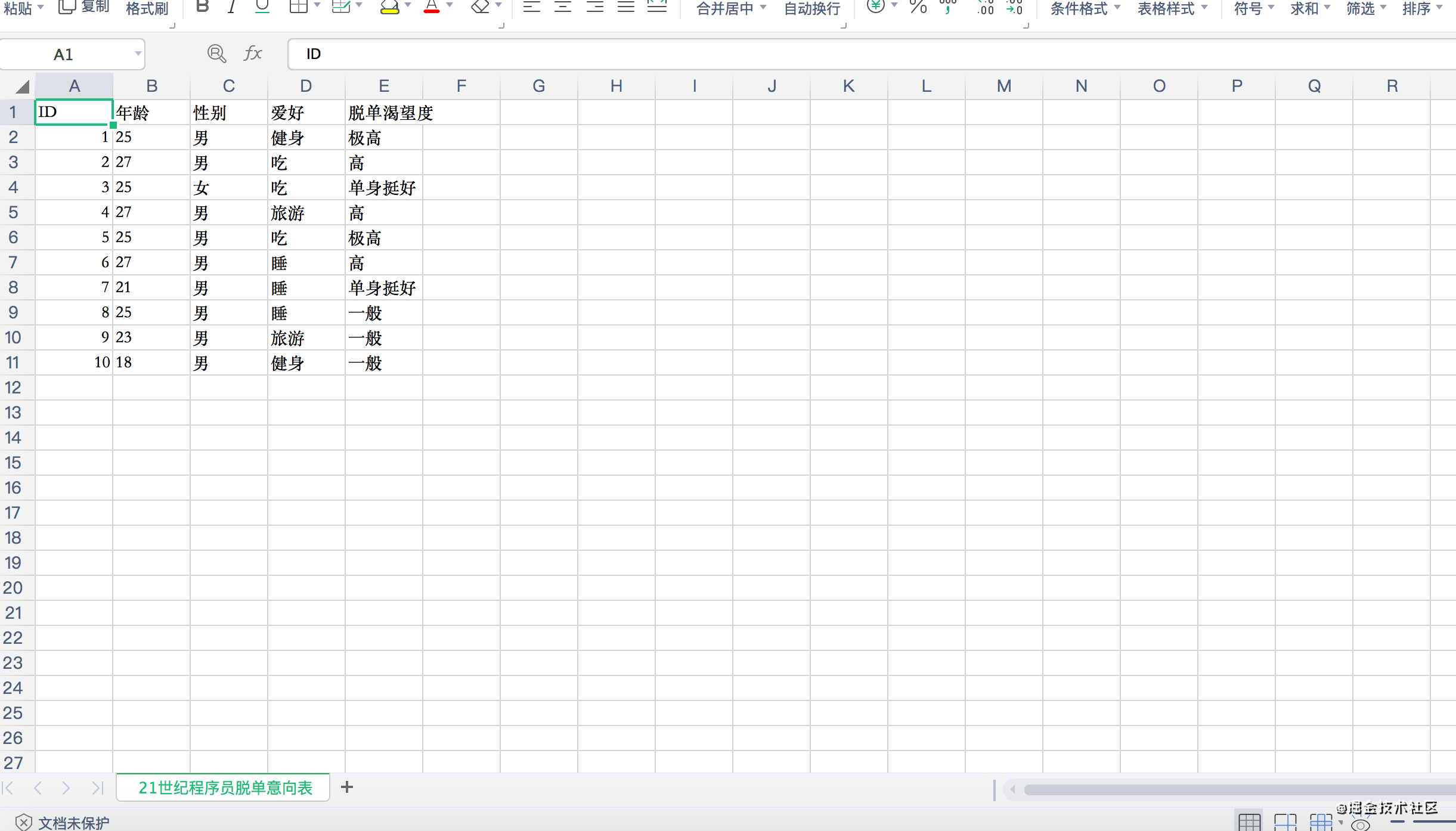## 前言

• 如何使用JavaScript实现前端导入和导出excel文件(H5编辑器实战复盘)
• 前端如何基于table中的数据一键生成多维度数据可视化分析报表
• 如何实现会员管理系统下的权限路由和权限菜单

• 使用JavaScript实现前端导入excel文件并自动生成可编辑的Table组件
• 使用JavaScript实现前端基于Table数据一键导出excel文件
• XLSXjs-export-excel基本使用

## 正文

### 1. 使用JavaScript实现前端导入excel文件并自动生成可编辑的Table组件

#### 1.2 实现一键导入excel文件并生成table表格

// 解析并提取excel数据
let data = e.target.result;
let workbook = XLSX.read(data, {type: 'binary'});
let sheetNames = workbook.SheetNames; // 工作表名称集合
let draftObj = {}
sheetNames.forEach(name => {
// 通过工作表名称来获取指定工作表
let worksheet = workbook.Sheets[name];
for(let key in worksheet) {
// v是读取单元格的原始值
if(key !== '!') {
if(draftObj[key]) {
draftObj[key].push(worksheet[key].v)
}else {
draftObj[key] = [worksheet[key].v]
}
}
}
});
// 生成ant-table支持的数据格式
let sourceData = Object.values(draftObj).map((item,i) => ({ key: i + '', name: item, value: item}))

#### 1.3 table表格的编辑功能实现

table表格的编辑功能实现其实也很简单, 我们只需要按照antd的table组件提供的自定义行和单元格的实现方式即可. antd官网上也有实现可编辑表格的实现方案, 如下:大家感兴趣的可以私下研究以下. 当然自己实现可编辑的表格也很简单, 而且有很多方式, 比如用columnrender函数来动态切换表格的编辑状态, 或者使用弹窗编辑等都是可以的.

#### 1.4 根据编辑的table数据动态生成图表

const data = [
{ genre: 'Sports', sold: 275 },
{ genre: 'Strategy', sold: 115 },
{ genre: 'Action', sold: 120 },
{ genre: 'Shooter', sold: 350 },
{ genre: 'Other', sold: 150 }
];

import { Chart } from '@antv/f2';
import React, { memo, useEffect, useRef } from 'react';
import ChartImg from '@/assets/chart.png';

import styles from './index.less';
import { IChartConfig } from './schema';

interface XChartProps extends IChartConfig {
isTpl: boolean;
}

const XChart = (props: XChartProps) => {
const { isTpl, data, color, size, paddingTop, title } = props;
const chartRef = useRef(null);
useEffect(() => {
if (!isTpl) {
const chart = new Chart({
el: chartRef.current || undefined,
pixelRatio: window.devicePixelRatio, // 指定分辨率
});

// step 2: 处理数据
const dataX = data.map(item => ({ ...item, value: Number(item.value) }));

// Step 2: 载入数据源
chart.source(dataX);

// Step 3：创建图形语法，绘制柱状图，由 genre 和 sold 两个属性决定图形位置，genre 映射至 x 轴，sold 映射至 y 轴
chart
.interval()
.position('name*value')
.color('name');

// Step 4: 渲染图表
chart.render();
}
}, [data, isTpl]);
return (
<div className={styles.chartWrap}>
<div className={styles.chartTitle} style={{ color, fontSize: size, paddingTop }}>
{title}
</div>
{isTpl ? <img src={ChartImg} alt="dooring chart" /> : <canvas ref={chartRef}></canvas>}
</div>
);
};

export default memo(XChart);

### 2. 使用JavaScript实现前端基于Table数据一键导出excel文件

#### 2.1 一键导出为excel实现效果以上就是用户基于后台采集到的数据, 一键导出excel文件的流程, 最后一张图是生成的excel文件在office软件中的呈现.

#### 2.2 使用javascript实现一键导出excel文件功能

• 自定义导出的excel文件名
• 自定义excel的过滤字段
• 自定义excel文件中每列的表头名称

const generateExcel = () => {
let option = {};  //option代表的就是excel文件
let dataTable = [];  //excel文件中的数据内容
let len = list.length;
if (len) {
for(let i=0; i<len; i++) {
let row = list[i];
let obj:any = {};
for(let key in row) {
if(typeof row[key] === 'object') {
let arr = row[key];
obj[key] = arr.map((item:any) => (typeof item === 'object' ? item.label : item)).join(',')
}else {
obj[key] = row[key]
}
}
dataTable.push(obj);  //设置excel中每列所获取的数据源
}
}
let tableKeys = Object.keys(dataTable);
option.fileName = tableName;  //excel文件名称
option.datas = [
{
sheetData: dataTable,  //excel文件中的数据源
sheetName: tableName,  //excel文件中sheet页名称
sheetFilter: tableKeys,  //excel文件中需显示的列数据
}
]
let toExcel = new ExportJsonExcel(option);  //生成excel文件
toExcel.saveExcel();  //下载excel文件
}

## 最后

github地址：H5在线编辑器H5-Dooring

## 更多推荐